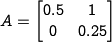# Exercise: Properties of linear discrete time systems

(diff) ← Older revision | Latest revision (diff) | Newer revision → (diff)

Consider the following discrete time systemwhere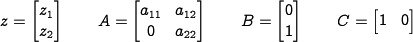In this problem, we will explore some of the properties of this discrete time system as a function of the parameters, the initial conditions, and the inputs.

1. Assume that the off diagonal elementand that there is no input,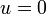. Write a closed form expression for the output of the system from a nonzero initial condition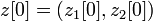and give conditions on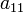and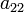under which the output gets smaller asgets larger.

2. Now assume thatand write a closed form expression for the response of the system from a nonzero initial conditions. Given a condition on the elements ofunder which the output gets smaller asgets larger.

3. Write a MATLAB program to plot the output of the system in response to a unit step input,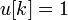,. Plot the response of your system withandgiven by